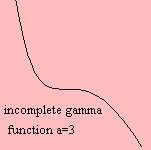# incomplete gamma function

## gammaThe incomplete gamma function Γ(a,x) is a variation on the gamma function.

An alternative incomplete gamma function can be defined as the integral from 0 to x. It may be clear that this is the same function, apart from a constant.

The function can be normalized to the regularized incomplete gamma function Q(a,x) defined by: Γ(a,x) / Γ(a).

The incomplete gamma function and its inverse are used in statistics.